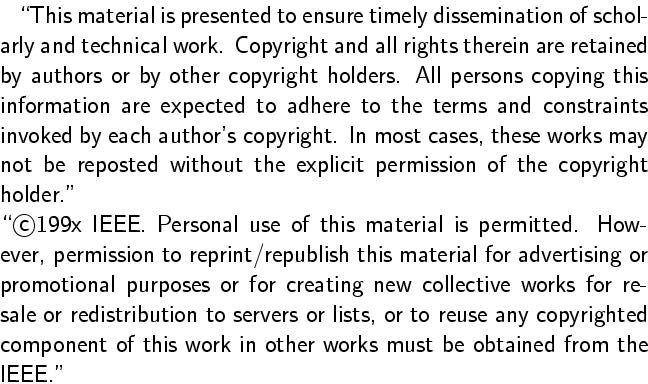Gunther Reißig and Ute Feldmann.
Computing the Generic Index of the Circuit Equations of Linear Active Networks.
Proc. 1996 Int. Symp. Circuits and Systems (ISCAS), Atlanta, GA, May 12-15, 1996, vol. III, pp. 190-193.
Full text. (Definitive publication; restricted access.)
Full text. (Free access.)Abstract:
In many problems, such as the analysis of electrical circuits, one has to deal with Differential-Algebraic Equations (DAE's). Knowing their generic index is of interest for practical and numerical as well as for theoretical reasons. In this paper we give an algorithm that determines the generic index of the Circuit Equations of linear active electrical networks in polynomial time from the network topology alone. It uses integer arithmetic and does neither require symbolic calculations nor real arithmetic.
BibTeX entry:
```@inproceedings{ReissigFeldmann96,
author = {Gunther Rei{\ss}ig and Ute Feldmann},
title = {Computing the generic index of the circuit equations of linear active networks},
booktitle = {Proc. 1996 IEEE Int. Symp. on Circuits and Systems (ISCAS), Atlanta, GA, } # may # { 12-15},
year = 1996,
volume = {III},
pages = {190-193},
doi = {10.1109/ISCAS.1996.541512}
}
```

Impressum und Haftungsausschluß